# Tutorial for WebbPSF: Computing Simulated Point Spread Functions for JWST¶

This tutorial will walk you through the basics of using the WebbPSF package to calculate PSFs for JWST. This focuses on the command-line/programming interface rather than the graphical interface. Let's begin.

First, we set up the notebook to display plots inline, and to plot images with the origin in the lower left.

In :
%pylab inline --no-import-all
matplotlib.rcParams['image.origin'] = 'lower'

# Ignore some unrelated deprecation warnings from current version of Matplotlib
import warnings
import matplotlib.cbook
warnings.filterwarnings("ignore",category=matplotlib.cbook.mplDeprecation)

Populating the interactive namespace from numpy and matplotlib


## Getting Started¶

We assume you have already installed webbpsf from PyPI or some other source::

    pip install webbpsf --upgrade
In :
import webbpsf


WebbPSF produces various log messages while it works, using Python's built-in logging mechanism. In order to see them, we need to set up a log handler that will display them on the screen. This is done using the setup_logging function.

In :
webbpsf.setup_logging()

WebbPSF log messages of level INFO and above will be shown.
WebbPSF log outputs will be directed to the screen.


We can also choose to save log outputs to a file, if that's desired.

In :
webbpsf.setup_logging(filename='my_log.txt')

WebbPSF log messages of level INFO and above will be shown.
WebbPSF log outputs will be directed to the screen.
WebbPSF log outputs will also be saved to file my_log.txt


## First PSFs¶

Now let's get started with some calculations. WebbPSF provides a set of five objects corresponding to JWST's four instruments plus the FGS. To calculate a PSF, we first instantiate one of these:

In :
nc = webbpsf.NIRCam()


And then call its calcPSF function. Note the log output describes various details of the calculation as it proceeds. The returned result is a fits HDUList object containing both the image data and its associated metadata in the header.

In :
psf = nc.calc_psf(nlambda=5, fov_arcsec=2)

[  poppy] No source spectrum supplied, therefore defaulting to 5700 K blackbody
[  poppy] Computing wavelength weights using synthetic photometry for F200W...
[  poppy] PSF calc using fov_arcsec = 2.000000, oversample = 4, number of wavelengths = 5
[webbpsf] Creating optical system model:
[  poppy] Initialized OpticalSystem: JWST+NIRCam
[  poppy] JWST Entrance Pupil: Loaded amplitude transmission from /Users/mperrin/software/webbpsf-data/jwst_pupil_RevW_npix1024.fits.gz
[  poppy] JWST Entrance Pupil: Loaded OPD from /Users/mperrin/software/webbpsf-data/NIRCam/OPD/OPD_RevW_ote_for_NIRCam_requirements.fits
[  poppy] The supplied pupil OPD is a datacube but no slice was specified. Defaulting to use slice 0.
[  poppy] Added pupil plane: JWST Entrance Pupil
[  poppy] Added coordinate inversion plane: OTE exit pupil
[  poppy] Added detector with pixelscale=0.0311 and oversampling=4: NIRCam detector
[  poppy] Calculating PSF with 5 wavelengths
[  poppy]  Propagating wavelength = 1.79059e-06 m
[  poppy]  Propagating wavelength = 1.89095e-06 m
[  poppy]  Propagating wavelength = 1.9913e-06 m
[  poppy]  Propagating wavelength = 2.09165e-06 m
[  poppy]  Propagating wavelength = 2.19201e-06 m
[  poppy]   Calculation completed in 0.256 s
[  poppy] PSF Calculation completed.
[  poppy] Calculating jitter using gaussian
[  poppy] Jitter: Convolving with Gaussian with sigma=0.007 arcsec
[  poppy]         resulting image peak drops to 0.944 of its previous value
[  poppy]  Adding extension with image downsampled to detector pixel scale.
[  poppy]  Downsampling to detector pixel scale, by 4


As you can see, the log output can be fairly verbose. This is often helpful in terms of understanding what's going on, but for purposes of this documentation it will make for easier reading to turn off display of informational messages:

In :
webbpsf.setup_logging('ERROR')

WebbPSF log messages of level ERROR and above will be shown.
WebbPSF log outputs will be directed to the screen.


We can display the PSF that we have just created:

In :
webbpsf.display_psf(psf)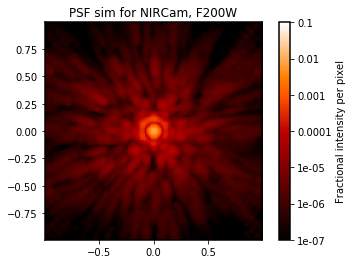The default behavior of WebbPSF is to compute oversampled PSFs (i.e. sampled more finely than the detector pixel scale) by a factor of 4. However, it also produces a version of the same PSF that has been downsampled onto the detector scale. This is saved as an image extension in the same result file:

In :
webbpsf.display_psf(psf,ext='DET_SAMP')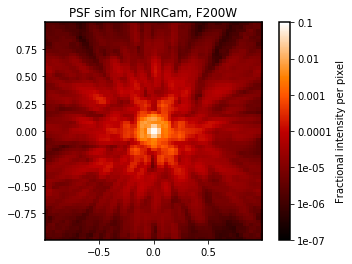Instruments have properties corresponding to their configurable options, typically the bandpass filter and optional image plane and pupil plane masks.

In :
nc.filter='F444W'
psf444 = nc.calc_psf(fov_arcsec=2)
webbpsf.display_psf(psf444)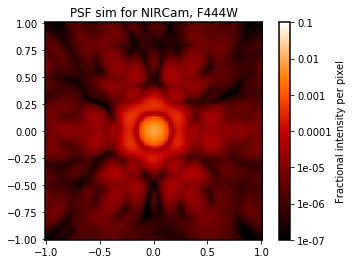In :
nc.filter='F212N'
psf212 = nc.calc_psf(fov_arcsec=3)
webbpsf.display_psf(psf212)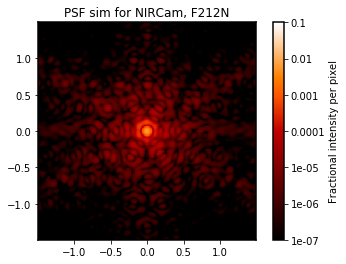More complicated instrumental configurations are available by setting the instrument’s attributes. For instance, one can create an instance of MIRI and configure it for coronagraphic observations, as follows. This also shows off the ability to display the intermediate optical planes during a calculation:

In :
# optional: make the plot bigger so we can see everything more easily
plt.figure(figsize=(14, 8))

miri = webbpsf.MIRI()
miri.filter = 'F1065C'
miri_coron = miri.calc_psf(display=True)
plt.savefig('fig_miri_coron_f1065c.png', dpi=100)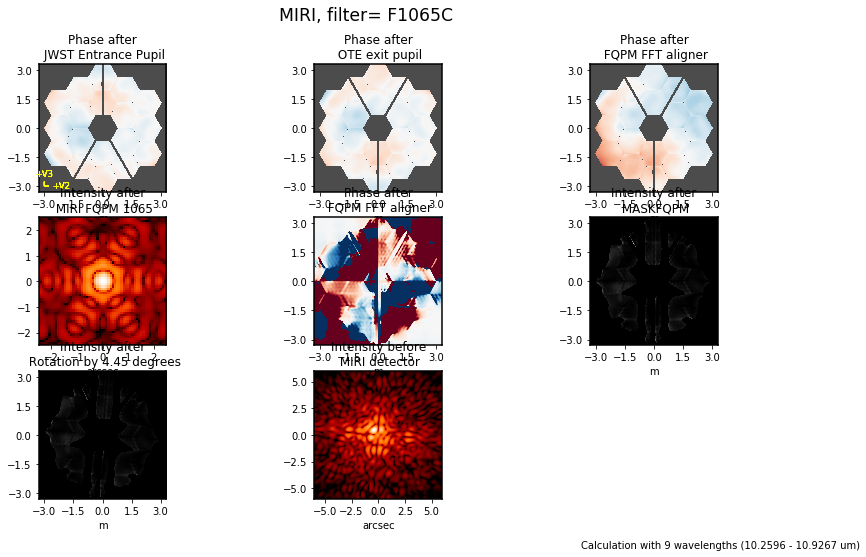## More Examples¶

Here are some other example calculations taken from the rest of the webbpsf documentation.

Some of these differs cosmetically from the code there: this notebook contains some extra function calls to set an aesthetically pleasing size for each plot, and to save the outputs to PNGs for inclusion in the documentation source code. These lines are left out of the example docs HTML page just to streamline it a bit.

In :
# Iterate over all instruments, calculating one example PSF for each
insts = ['NIRCam','NIRCam','NIRSpec','NIRISS', 'MIRI', 'FGS']
filts = ['F210M', 'F444W', 'F110W', 'F380M', 'F1000W', 'FGS']

psfs = {}
for i, (instname, filt) in enumerate(zip(insts, filts)):
inst = webbpsf.Instrument(instname)
inst.filter = filt
psf = inst.calc_psf(fov_arcsec=5.0)
psfs[instname+filt] = psf
print(inst)

<JWST: NIRCam>
<JWST: NIRCam>
<JWST: NIRSpec>
<JWST: NIRISS>
<JWST: MIRI>
<JWST: FGS>

In :
# Now make a nice plot of them
plt.figure(figsize=(16*0.75,3*0.75))
for i, (instname, filt) in enumerate(zip(insts, filts)):
ax = plt.subplot(1,6,1+i)
webbpsf.display_psf(psfs[instname+filt], colorbar=False, title=instname+" "+filt, vmax=0.03, imagecrop=5)
if i > 0:
ax.yaxis.set_visible(False)
plt.tight_layout()
plt.savefig('fig_instrument_comparison.png', dpi=150)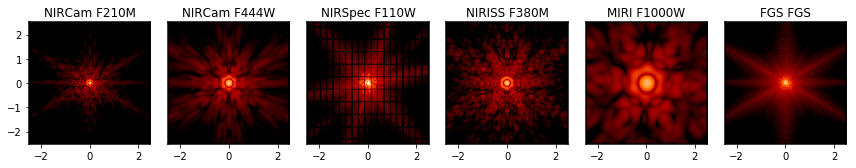In :
# Example plot of F200W calculation
plt.figure(figsize=(10,5))
nc = webbpsf.NIRCam()
nc.filter =  'F200W'
nc.calc_psf(display=True)
plt.savefig('fig1_nircam_f200w.png', dpi=120)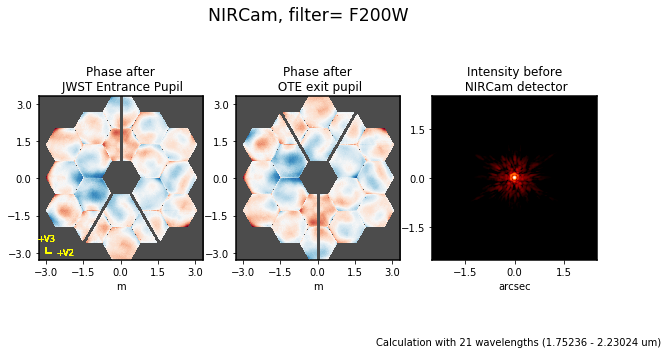## Displaying a PSF as an image and as an encircled energy plot¶

In :
#create a NIRCam instance and calculate a PSF for F210M
nircam = webbpsf.NIRCam()
nircam.filter = 'F210M'
psf210 = nircam.calcPSF(oversample=2)

# display the PSF and plot the encircled energy
plt.figure(figsize=(9,5))
plt.subplot(1,2,1)
webbpsf.display_psf(psf210, colorbar_orientation='horizontal')
axis2 = plt.subplot(1,2,2)
webbpsf.display_ee(psf210, ax=axis2)

psf210.writeto('nircam_F210M.fits', clobber=True)
plt.savefig('fig_example_plot_nircam_f210m.png')

WARNING: AstropyDeprecationWarning: "clobber" was deprecated in version 2.0 and will be removed in a future version. Use argument "overwrite" instead. [__main__]
[astropy] AstropyDeprecationWarning: "clobber" was deprecated in version 2.0 and will be removed in a future version. Use argument "overwrite" instead.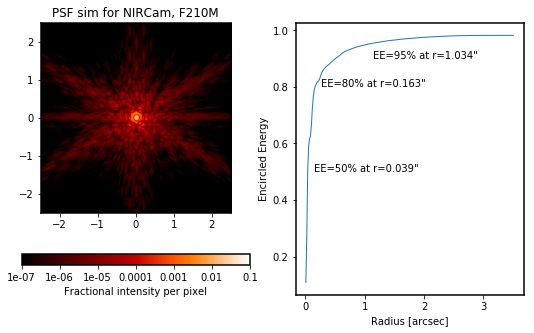## Jupyter Notebook Widget Interface¶

WebbPSF includes an interactive Jupyter notebook interface, which enables quick exploration of PSFs from changing some of the basic options such as filters and image or pupil masks. More complicated calculations need the Python API, but this can be useful for quick calculations.

In :
webbpsf.show_notebook_interface('nircam')

In [ ]:

`